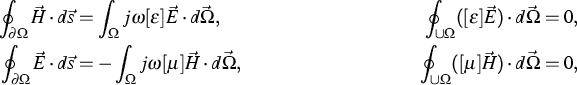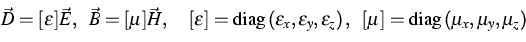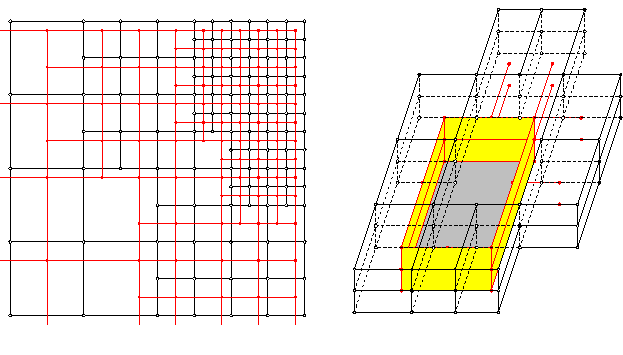[Next]: pdelib Algorithms and software
 [Up]: Project descriptions
 [Previous]: Large-scale static and dynamic process simulation
[Contents]   [Index]

## Simulation of microwave and semiconductor laser structures

Collaborator: G. Hebermehl , J. Schefter , R. Schlundt , F.-K. Hübner

Cooperation with: W. Heinrich, M. Kunze, T. Tischler, H. Zscheile (Ferdinand-Braun-Institut für Höchstfrequenztechnik, Berlin (FBH))

Supported by: Ferdinand-Braun-Institut für Höchstfrequenztechnik (FBH)

Description:

The electric properties of circuits are described in terms of their scattering matrix using a three-dimensional boundary value problem for Maxwell's equations. The computational domain is truncated by electric or magnetic walls; open structures are treated using the Perfectly Matched Layer (PML) absorbing boundary condition.

The subject under investigation is about passive structures of arbitrary geometry. They are connected to the remaining circuit by transmission lines. Ports are defined on the waveguides. In order to characterize their electrical behavior the transmission lines are assumed to be infinitely long and longitudinally homogeneous.

The boundary value problemis solved by means of a finite-volume scheme in the frequency domain. This results in a two-step procedure: an eigenvalue problem for complex matrices and the solution of large-scale systems of linear algebraic equations with indefinite symmetric complex matrices.

Due to the fact that only small fractions of a circuit can be simulated, the pressure for larger problem sizes and shorter computing times is evident. Thus, in the period under report, studies were continued in the following areas:

(1) Eigenmode problem : Only a few modes of smallest attenuation are able to propagate and have to be taken into consideration. Using a conformal mapping between the plane of propagation constants and the plane of eigenvalues, the task is to compute all eigenmodes in a region, bounded by two parabolas. The region is covered by a number of overlapping circles. The eigenmodes in these circles are found solving a sequence of eigenvalue problems of modified matrices with the aid of the invert mode of the Arnoldi iteration using shifts. The method, developed initially for a reliable calculation of all complex eigenvalues from microwave structure computations , is expanded to meet the very special requirements of optoelectronic structure calculations. Relatively large cross sections and highest frequencies yield increased dimensions and numbers of eigenvalue problems. Using the results of a coarse grid calculation within the final fine grid calculation yields a remarkable reduced numerical effort. The use of the new linear sparse solver PARDISO  (see page) rather than UMFPACK , and two levels of parallelization result in an additional speed-up of computation time.

(2) Boundary value problem : The electromagnetic fields are computed by the solution of large-scale systems of linear equations with indefinite complex symmetric coefficient matrices. In general, these matrix problems have to be solved repeatedly for different right-hand sides, but with the same coefficient matrix. The number of right-hand sides depends on the number of ports and modes. The systems of linear equations are solved using a block Krylov subspace iterative method. Independent set orderings, Jacobi and SSOR preconditioning techniques are applied to reduce the dimension and the number of iterations . In comparison to the simple lossy case the number of iterations of Krylov subspace methods increases significantly in the presence of Perfectly Matched Layers. This growth could be further reduced by applying suitable grids and PML. The speed of convergence depends on the relations of the edges in an elementary cell of the nonequidistant rectangular grid in this case. The best results can be obtained using nearly cubic cells grids. Moreover, overlapping PML conditions on the corners downgrade the properties of the coefficient matrix, and should be avoided.

(3) Consistent subgridding scheme: For the above-mentioned methods the geometry to be analyzed is subdivided into elementary rectangular cells using three-dimensional nonequidistant rectangular cells. Applying rectangular grids, a mesh refinement in one point results in an accumulation of elementary cells in all coordinate directions even though generally the refinement is needed only in certain regions. Due to the high spatial resolution required, CPU time and storage requirements are very high. A general approach to handle small structure details is to introduce local mesh refinement () with hanging nodes. Energy and divergence conservation have to be conserved throughout the discretization process for Maxwell's equations. Maxwell's grid equations have been derived for some kinds of submeshes.

(4) Tetrahedral grids : Rectangular grids are not well suitable for the treatment of curved and non-rectangular boundaries. Thus, supported by FBH, the development of a finite-volume method was continued by using unstructured grids (tetrahedrals, see page) for the boundary value problem.

The new research results have been published in  - .

References:

1. K. BEILENHOFF, W. HEINRICH, H.L. HARTNAGEL, Improved finite-difference formulation in frequency domain for three-dimensional scattering problems, IEEE Transactions on Microwave Theory and Techniques, 40 (1992), pp. 540-546.
2. A. CHRIST, H.L. HARTNAGEL, Three-dimensional finite-difference method for the analysis of microwave-device embedding, IEEE Transactions on Microwave Theory and Techniques, 35 (1987), pp. 688-696.
3. T.A. DAVIS, I.S. DUFF, A combined unifrontal/multifrontal method for unsymmetric sparse matrices, ACM Trans. Math. Software, 25 (1999), pp. 1-19.
4. G. HEBERMEHL, F.-K. HÜBNER, R. SCHLUNDT, TH. TISCHLER, H. ZSCHEILE, W. HEINRICH, Numerical simulation of lossy microwave transmission lines including PML, in: Scientific Computing in Electrical Engineering, U. van Rienen, M. Günther, D. Hecht, eds., vol. 18 of Lecture Notes Comput. Sci. Eng., Springer, Berlin, 2001, pp. 267-275.
5., Eigen mode computation of microwave and laser structures including PML, to appear in: Mathematics in Industry, Scientific Computing in Electrical Engineering, W.H.A. Schilders, S.H.M.J. Houben, E.J.W. ter Maten, eds., Springer.
6., Perfectly matched layers in transmission lines, to appear in: Numerical Mathematics and Advanced Applications, ENUMATH 2001, F. Brezzi, A. Buffa, S. Corsaro, A. Murli, eds., Springer Verlag Italia Srl.
7., Eigen mode computation of microwave and laser structures including PML, in: SCEE-2002, Scientific Computing in Electrical Engineering, June 23-28, 2002, Eindhoven, The Netherlands, Book of Reviewed Abstracts, W.H.A. Schilders, S.H.M.J. Houben, E.J.W. ter Maten, eds., Eindhoven University of Technology, Philips Research Laboratories Eindhoven, 2002, pp. 95-96.
8., Numerical techniques in the simulation of microwave and laser structures including PML, in: Proceedings of the 10th International IGTE Symposium Numerical Field Calculation in Electrical Engineering, September 16-18, 2002, Graz, Austria, Verlag der Technischen Universität Graz, Austria, 2002, pp. 1-13.
CD-ROM, ISBN 3-901351-65-5.
9. G. HEBERMEHL, On the computation of propagating modes for microwave and optoelectronic devices including PML, in: Annual Scientific Conference, Gesellschaft für Angewandte Mathematik und Mechanik e.V. (GAMM), University of Augsburg, Germany, March 25-28, 2002, Book of Abstracts, Springer, Heidelberg, 2002, pp. 59-60.
10. G. HEBERMEHL, R. SCHLUNDT, TH. TISCHLER, H. ZSCHEILE, W. HEINRICH, F.-K. HÜBNER, Simulation of microwave and semiconductor laser structures including absorbing boundary conditions. WIAS Preprint no. 803, 2002.
11. J. SCHEFTER, Diskretisierung der Maxwell'schen Gleichungen auf Tetraedergittern (Studie), 2001, 72 p.
12. O. SCHENK, K. GÄRTNER, W. FICHTNER, Efficient sparse LU factorization with left-right looking strategy on shared memory multiprocessors, BIT, 40 (2000), pp. 158-176.
13. R. SCHLUNDT, G. HEBERMEHL, F.-K. HÜBNER, W. HEINRICH, H. ZSCHEILE, Iterative solution of systems of linear equations in microwave circuits using a block quasi-minimal residual algorithm, in: Scientific Computing in Electrical Engineering, U. van Rienen, M. Günther, D. Hecht, eds., vol. 18 of Lecture Notes Comput. Sci. Eng., Springer, Berlin, 2001, pp. 325-333.
14. P. THOMA, T. WEILAND, A consistent subgridding scheme for the finite difference time domain method, Internat. J. Numer. Modelling, 9 (1996), pp. 359-374.[Next]: pdelib Algorithms and software
 [Up]: Project descriptions
 [Previous]: Large-scale static and dynamic process simulation
[Contents]   [Index]

LaTeX typesetting by I. Bremer
5/16/2003